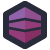The HP3000 was the first mini-computer that supported time-sharing. It launched in 1972, and HP didn't end-of-life it until 2010, and there are still third-party vendors supporting them.

Leonora's submission is some code she encountered a number of years ago, but not as many as you might think. It's in Pascal, and it's important to note that this version of Pascal definitely has bitwise operators. But, if you're programming on a 40 year old minicomputer, maybe you couldn't do an Internet search, and maybe Steve from down the hall had bogarted the one manual HP provided for the computer so you can't look it up because "he's using it for reference."

So you end up doing your best with no idea:

``````FUNCTION BITON(A , B : INTEGER): BOOLEAN;
VAR
C : INTEGER;
BEGIN
CASE A OF
15 : C:=1;
14 : C:=2;
13 : C:=4;
12 : C:=8;
11 : C:=16;
10 : C:=32;
9 : C:=64;
8 : C:=128;
7 : C:=256;
6 : C:=512;
5 : C:=1024;
4 : C:=2048;
3 : C:=4096;
2 : C:=8192;
1 : C:=16384;
0 : C:=32768;
OTHERWISE
BITON:=FALSE;
END;
IF ((B DIV C) MOD 2) = 1 THEN
BITON:=TRUE
ELSE
BITON:=FALSE;
END;
``````

One thing I appreciate about Pascal code is that, even if you haven't touched the language since 1998 when you were the last class in your high school to learn Pascal instead of C++, it's incredibly readable. This method is very clear about what it does: it maps bits to powers of two, and then checks via division and modulus if that bit is set. It's, in some ways, the most obvious way to implement a bit check if you didn't know anything about bitwise operations in your language.

This `BITON` function lays the foundation for an entire family of bitwise operations, re-implemented from scratch. You want to set bits individually? You already know how it works.

``````FUNCTION SETBITON(A, B : INTEGER) : INTEGER;
VAR
C : INTEGER;
BEGIN
CASE A OF
15 : C:=1;
14 : C:=2;
13 : C:=4;
12 : C:=8;
11 : C:=16;
10 : C:=32;
9 : C:=64;
8 : C:=128;
7 : C:=256;
6 : C:=512;
5 : C:=1024;
4 : C:=2048;
3 : C:=4096;
2 : C:=8192;
1 : C:=16384;
0 : C:=32768;
OTHERWISE
C:=0;
END;
IF NOT BITON(A,B) THEN
SETBITON:=B + C
ELSE
SETBITON:=B;
END;

FUNCTION SETBITOFF(A, B : INTEGER) : INTEGER;
VAR
C : INTEGER;
BEGIN
CASE A OF
15 : C:=1;
14 : C:=2;
13 : C:=4;
12 : C:=8;
11 : C:=16;
10 : C:=32;
9 : C:=64;
8 : C:=128;
7 : C:=256;
6 : C:=512;
5 : C:=1024;
4 : C:=2048;
3 : C:=4096;
2 : C:=8192;
1 : C:=16384;
0 : C:=32768;
OTHERWISE
C:=0;
END;
IF BITON(A,B) THEN
SETBITOFF:=B - C
ELSE
SETBITOFF:=B;
END;
``````

The same pattern, complete with 16 bits hand-coded, and a check: if the bit is on, we add or subtract a value to set it off.

And, if you want an `and` operator, why, that's just going to call those methods:

``````FUNCTION LAND(A,B : INTEGER) : INTEGER;
VAR
I : INTEGER;
BEGIN
I:=0;
REPEAT
IF BITON(I,A) THEN
IF BITON(I,B) THEN
A:=SETBITON(I,A)
ELSE
A:=SETBITOFF(I,A)
ELSE
A:=SETBITOFF(I,A);
I:=I + 1;
UNTIL I > 15;
LAND:=A;
END;
``````

Again, I want to stress, while different Pascal compilers have different implementations of bitwise operations, this version absolutely had bitwise operations. As do most of them. The key difference is whether or not it supports C-style `<<`/`>>` operators, or uses a more Pascal-flavored `shl`/`shr`.

This developer obviously didn't know about them, and didn't know about the right way to do exponentiation either, which would make those giant case statements go away. Not as well as bitshifting, but still, away. But they clearly did their best- the code is readable, clear, and obvious in how it functions. Just, y'know, not as obvious as using the built-ins.[Advertisement] Otter - Provision your servers automatically without ever needing to log-in to a command prompt. Get started today!